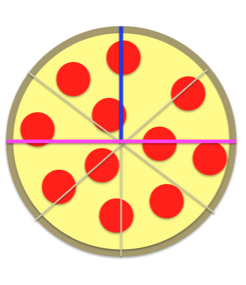# Perimeter and Area of a Semicircle Problems & Flashcards

Perimeter and Area of a Semicircle Problems & Flashcards
1/17 (missed) 0 0
Create Your Account To Continue Studying

As a member, you'll also get unlimited access to over 79,000 lessons in math, English, science, history, and more. Plus, get practice tests, quizzes, and personalized coaching to help you succeed.

Try it risk-free for 30 days. Cancel anytime
The radius is 1/2 of the diameter. The diameter is the line that runs across the midpoint.
Got it
Find the area of the semicircle with a radius of 9CM.
Answer: 127.23CM. Use this equation; Pi x r^2 / 2 = Area of a semicircle ('r' = radius, 3.14 = Pi)
Got it
Find the area of a semicircle with a radius of 5CM.
Answer: 39.27CM. Use this equation; Pi x r^2 / 2 = Area of a semicircle ('r' = radius, 3.14 = Pi)
Got it
Name this equation and its parts: Pi x r^2 / 2
Pi x r^2 / 2 is the equation used to find the area of a semicircle. ('r' = radius, 3.14 = Pi)
Got it
Find the perimeter of a semicircle with the radius of 3CM.
Answer: 12.42CM. Use the equation; Pi x r + d= Perimeter of a semicircle (3.14 = Pi, 'r' = radius, 'd' = diameter)
Got it
Find the perimeter of a semicircle with a radius of 8CM.
Answer: 41.12CM. Use the equation; Pi x r + d = perimeter of a semicircle (3.14 = Pi, 'r' = radius, 'd' = diameter)
Got it
Name this equation and its parts: Pi x r + d
Pi * r + d is the equation to find the perimeter of a semicircle. 'r' = radius, 'd' = diameter, Pi = 3.14.
Got it
Semicircle
A semicircle is 1/2 of a circle.Got it

or choose a specific lesson: See all lessons in this chapter
17 cards in set

## Flashcard Content Overview

Use this flashcard set to test your knowledge and gauge your understanding of semicircles! This flashcard set covers an overview of common terminology and equations. You will discover what a semicircle is and how these equations can be used to find the area and perimeter of any semicircle.

Front
Back
Semicircle
A semicircle is 1/2 of a circle.Name this equation and its parts: Pi x r + d
Pi * r + d is the equation to find the perimeter of a semicircle. 'r' = radius, 'd' = diameter, Pi = 3.14.
Find the perimeter of a semicircle with a radius of 8CM.
Answer: 41.12CM. Use the equation; Pi x r + d = perimeter of a semicircle (3.14 = Pi, 'r' = radius, 'd' = diameter)
Find the perimeter of a semicircle with the radius of 3CM.
Answer: 12.42CM. Use the equation; Pi x r + d= Perimeter of a semicircle (3.14 = Pi, 'r' = radius, 'd' = diameter)
Name this equation and its parts: Pi x r^2 / 2
Pi x r^2 / 2 is the equation used to find the area of a semicircle. ('r' = radius, 3.14 = Pi)
Find the area of a semicircle with a radius of 5CM.
Answer: 39.27CM. Use this equation; Pi x r^2 / 2 = Area of a semicircle ('r' = radius, 3.14 = Pi)
Find the area of the semicircle with a radius of 9CM.
Answer: 127.23CM. Use this equation; Pi x r^2 / 2 = Area of a semicircle ('r' = radius, 3.14 = Pi)
The radius is 1/2 of the diameter. The diameter is the line that runs across the midpoint.
Area
The area is the space within the semicircle.
Perimeter
The perimeter, or circumference, is the total length of all the sides.
Circumference
The circumference, or perimeter, is the total length of all the sides.
Find the perimeter of a semicircle with a diameter of 4 inches.
Answer: 10.28 Inches. Use the equation; Pi x r + d= Perimeter of a semicircle (3.14 = Pi, 'r' = radius, 'd' = diameter)
Find the perimeter of a semicircle with a diameter of 6 Inches.
Answer: 15.42 Inches. Use the equation; Pi x r + d= Perimeter of a semicircle (3.14 = Pi, 'r' = radius, 'd' = diameter)
The blue line represents the _____.The pink line represents the _____.The diameter
The purple color represents the _____.The area
The black line represents the _____.The perimeter or circumference

To unlock this flashcard set you must be a Study.com Member.